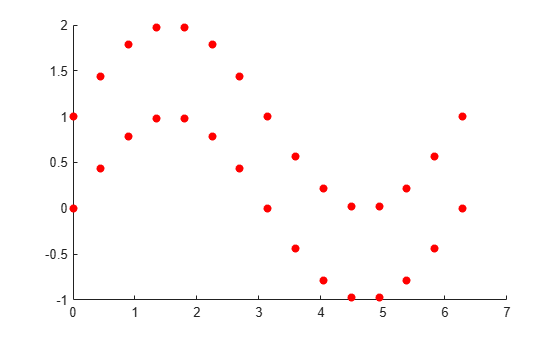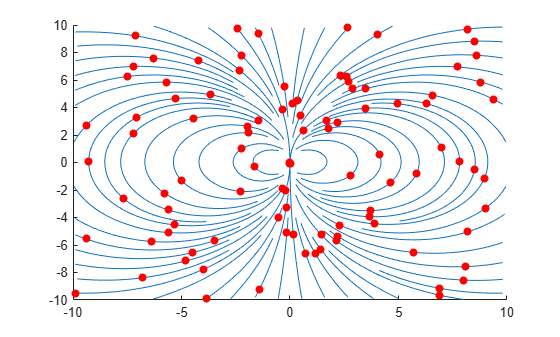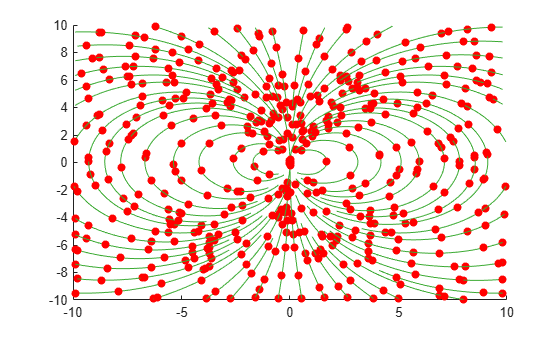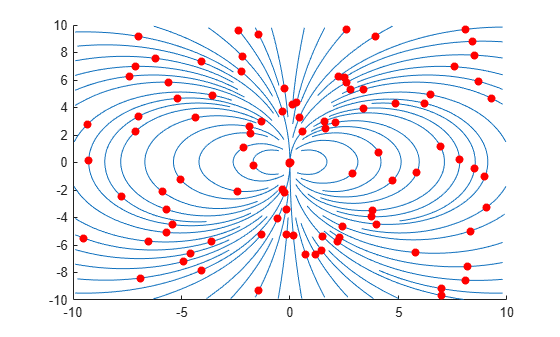# streamparticles

Plot stream particles

## Syntax

``streamparticles(verts)``
``streamparticles(verts,n)``
``streamparticles(___,Name,Value)``
``streamparticles(target,___)``
``lineobj = streamparticles(___)``

## Description

example

````streamparticles(verts)` plots stream particles at each vertex of the streamlines of a vector field. Stream particles can show the position and velocity of a streamline.```

example

````streamparticles(verts,n)` uses `n` to determine how many stream particles to plot. `n` can represent the number of particles or a percentage of streamline vertices. You can use the `ParticleAlignment` name-value argument with this syntax to change how `streamparticles` uses `n`.```

example

````streamparticles(___,Name,Value)` modifies stream particle animation and appearance by using one or more name-value arguments to set properties. For example, you can set the shape of the particles using the `Marker` property. Specify name-value arguments after all the arguments in any of the previous syntaxes. For a list of properties, see Line Properties.```
````streamparticles(target,___)` uses the target object to create the stream particles plot. If the target object is an `Axes` object, then MATLAB® plots into the specified axes instead of the current axes (`gca`). If the target object is a `Line` object, then the line properties are updated to create the stream particles instead of creating a new `Line` object.```
````lineobj = streamparticles(___)` returns the `Line` object that contains all particle vertices.```

## Examples

collapse all

Create vertices for two streamlines, specified as x- and y-coordinates in 15-by-2 matrices. Define the vertex input to `streamparticles` as a cell array, where each element represents one streamline.

```x = linspace(0,2*pi,15); y1 = sin(x); y2 = sin(x) + 1; s1 = [x;y1]'; s2 = [x;y2]'; verts = {s1,s2};```

Plot particles at each vertex of the streamlines.

`streamparticles(verts);`Define a vector field using position and velocity matrices. Use `streamslice` to generate the vertices of the streamlines in the vector field, and store the vertices in `verts`.

```[x,y] = meshgrid(-10:10); u = 2.*x.*y; v = y.^2 - x.^2; [verts,~] = streamslice(x,y,u,v);```

Plot the streamlines with `streamline`. Then, plot 100 particles with `streamparticles`. By default, the particles are spaced evenly over all the vertices.

```streamline(verts); streamparticles(verts,100);```For the same vector field, plot five particles evenly over the streamline with the most vertices by setting the `ParticleAlignment` property to `"on"`. The `streamparticles` function uses that spacing to plot particles on the remaining streamlines.

```streamline(verts); streamparticles(verts,5,"ParticleAlignment","on");```For the same vector field, plot 5% of the streamline vertices as particles.

```streamline(verts); streamparticles(verts,0.05);```Create the vertices for a vector field, and plot its streamlines and stream particles. Animate the particles for five iterations at 30 frames per second by setting the `Animate` and `FrameRate` properties, respectively.

```[x,y] = meshgrid(-10:10); u = 2.*x.*y; v = y.^2 - x.^2; [verts,~] = streamslice(x,y,u,v); streamline(verts); streamparticles(verts,100,"Animate",5,"FrameRate",30);```Create the vertices for a vector field, and plot its streamlines and stream particles. Use green asterisk markers for the particles by setting the `Marker` and M`arkerEdgeColor` properties.

```[x,y] = meshgrid(-10:10); u = 2.*x.*y; v = y.^2 - x.^2; [verts,~] = streamslice(x,y,u,v); streamline(verts); streamparticles(verts,150,"Marker","*","MarkerEdgeColor",[0 0.5 0]);```## Input Arguments

collapse all

Streamline coordinate data, specified as a cell array (as returned by `stream2`, `stream3`, or `streamslice`). Each element of the cell array is a matrix of 2-D or 3-D vertices that defines one streamline, where each row represents the coordinates of one particle.

Number of stream particles, specified as a positive value.

• If `n` is greater than `1`, `streamparticles` plots approximately `n` particles.

• If `n` is less than or equal to `1`, `streamparticles` plots a percentage of the vertices as particles. For example, if `n` is `0.2`, `streamparticles` plots approximately 20% of the vertices.

By default, `streamparticles` plots the number of particles determined by `n` evenly over all streamline vertices. However, if you set the `ParticleAlignment` property to `"on"`, `streamparticles` plots the particles evenly over the streamline with the most vertices and then uses that particle spacing for the other streamlines.

Target object, specified as an `Axes` or a `Line` object.

• `Axes` object — `streamparticles` plots into the specified axes instead of the current axes.

• `Line` object — The line properties are updated to create the stream particles instead of creating a new `Line` object.

You can display a legend in a `streamparticles` plot by creating a `Line` object, calling `legend`, and then calling `streamparticles` with that line as the target object.

### Name-Value Arguments

Specify optional pairs of arguments as `Name1=Value1,...,NameN=ValueN`, where `Name` is the argument name and `Value` is the corresponding value. Name-value arguments must appear after other arguments, but the order of the pairs does not matter.

Example: `streamparticles(verts,MarkerFaceColor="blue")` specifies a blue marker for the stream particles.

Before R2021a, use commas to separate each name and value, and enclose `Name` in quotes.

Example: `streamparticles(verts,"MarkerFaceColor","blue")` specifies a blue marker for the stream particles.

Note

The line properties listed here are only a subset of properties that can change particle appearance. For a complete list, see Line Properties.

Number of animation iterations, specified as a nonnegative integer. By default, the value of `Animate` is `0`, which specifies no stream particle motion. If the value is `Inf`, the animation continues until you press Ctrl+C.

Animation frames per second, specified as a nonnegative integer. By default, the value of `FrameRate` is `Inf`, which draws the animation as fast as possible given the limitations of the machine running `streamparticles`.

Alignment of particles on streamlines, specified as `"off"` or `"on"`.

• If `ParticleAlignment` is `"off"`, `n` determines the number of particles plotted, evenly spaced over all streamline vertices.

• If `ParticleAlignment` is `"on"`, `n` determines the number of particles plotted, evenly spaced over the streamline with the most vertices. The `streamparticles` function uses this spacing to plot particles on the remaining streamlines.

Marker symbol, specified as one of the values listed in this table. By default, particles are displayed as circles. If the marker symbol does not have a face, for instance, `"*"`, the marker edge color must be specified.

MarkerDescriptionResulting Marker
`"o"`Circle`"+"`Plus sign`"*"`Asterisk`"."`Point`"x"`Cross`"_"`Horizontal line`"|"`Vertical line`"square"`Square`"diamond"`Diamond`"^"`Upward-pointing triangle`"v"`Downward-pointing triangle`">"`Right-pointing triangle`"<"`Left-pointing triangle`"pentagram"`Pentagram`"hexagram"`Hexagram`"none"`No markersNot applicable

Marker outline color, specified as `"none"`, an RGB triplet, a hexadecimal color code, a color name, or a short name. By default, there is no marker outline color.

RGB triplets and hexadecimal color codes are useful for specifying custom colors.

• An RGB triplet is a three-element row vector whose elements specify the intensities of the red, green, and blue components of the color. The intensities must be in the range `[0,1]`; for example,``` [0.4 0.6 0.7]```.

• A hexadecimal color code is a character vector or a string scalar that starts with a hash symbol (`#`) followed by three or six hexadecimal digits, which can range from `0` to `F`. The values are not case sensitive. Thus, the color codes `"#FF8800"`, `"#ff8800"`, `"#F80"`, and `"#f80"` are equivalent.

Alternatively, you can specify some common colors by name. This table lists the named color options, the equivalent RGB triplets, and hexadecimal color codes.

Color NameShort NameRGB TripletHexadecimal Color CodeAppearance
`"red"``"r"``[1 0 0]``"#FF0000"``"green"``"g"``[0 1 0]``"#00FF00"``"blue"``"b"``[0 0 1]``"#0000FF"``"cyan"` `"c"``[0 1 1]``"#00FFFF"``"magenta"``"m"``[1 0 1]``"#FF00FF"``"yellow"``"y"``[1 1 0]``"#FFFF00"``"black"``"k"``[0 0 0]``"#000000"``"white"``"w"``[1 1 1]``"#FFFFFF"`Here are the RGB triplets and hexadecimal color codes for the default colors MATLAB uses in many types of plots.

`[0 0.4470 0.7410]``"#0072BD"``[0.8500 0.3250 0.0980]``"#D95319"``[0.9290 0.6940 0.1250]``"#EDB120"``[0.4940 0.1840 0.5560]``"#7E2F8E"``[0.4660 0.6740 0.1880]``"#77AC30"``[0.3010 0.7450 0.9330]``"#4DBEEE"``[0.6350 0.0780 0.1840]``"#A2142F"`Marker fill color, specified as `"red"`, `"none"`, an RGB triplet, a hexadecimal color code, a color name, or a short name. By default, the marker fill color is red.

RGB triplets and hexadecimal color codes are useful for specifying custom colors.

• An RGB triplet is a three-element row vector whose elements specify the intensities of the red, green, and blue components of the color. The intensities must be in the range `[0,1]`; for example,``` [0.4 0.6 0.7]```.

• A hexadecimal color code is a character vector or a string scalar that starts with a hash symbol (`#`) followed by three or six hexadecimal digits, which can range from `0` to `F`. The values are not case sensitive. Thus, the color codes `"#FF8800"`, `"#ff8800"`, `"#F80"`, and `"#f80"` are equivalent.

Alternatively, you can specify some common colors by name. This table lists the named color options, the equivalent RGB triplets, and hexadecimal color codes.

Color NameShort NameRGB TripletHexadecimal Color CodeAppearance
`"red"``"r"``[1 0 0]``"#FF0000"``"green"``"g"``[0 1 0]``"#00FF00"``"blue"``"b"``[0 0 1]``"#0000FF"``"cyan"` `"c"``[0 1 1]``"#00FFFF"``"magenta"``"m"``[1 0 1]``"#FF00FF"``"yellow"``"y"``[1 1 0]``"#FFFF00"``"black"``"k"``[0 0 0]``"#000000"``"white"``"w"``[1 1 1]``"#FFFFFF"`Here are the RGB triplets and hexadecimal color codes for the default colors MATLAB uses in many types of plots.

`[0 0.4470 0.7410]``"#0072BD"``[0.8500 0.3250 0.0980]``"#D95319"``[0.9290 0.6940 0.1250]``"#EDB120"``[0.4940 0.1840 0.5560]``"#7E2F8E"``[0.4660 0.6740 0.1880]``"#77AC30"``[0.3010 0.7450 0.9330]``"#4DBEEE"``[0.6350 0.0780 0.1840]``"#A2142F"`## Version History

Introduced before R2006a